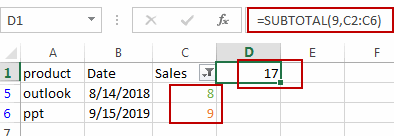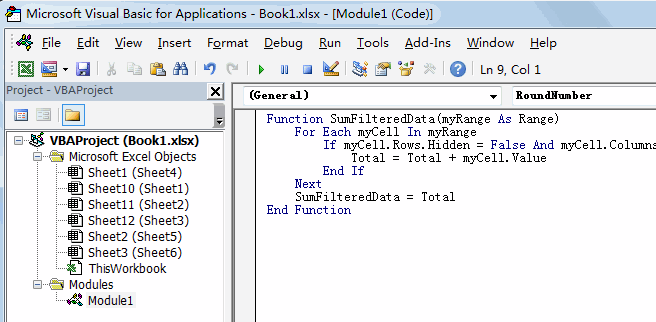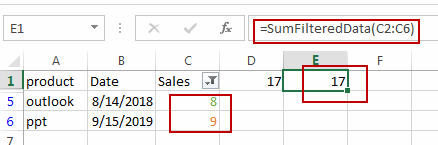# How to Sum Only Filtered Data or Visible Cell Values in Excel

This post will guide you how to sum only visible cell values in Excel. How do I sum only visible cell values in a selected range with a formula in Excel. How to sum only visible rows when filtering data in your worksheet with VBA code in Excel.

## Sum Filtered Data Only with Formula

Assuming that you have a list of data in range A1:C6, which contain sales s data. And you have filtered out the data that is greater than 5. Then you need to sum all filtered data only or all visible values without hidden values. How to achieve it. You can use a formula based on the SUBTOTAL function to achieve the result. Like this:

`=SUBTOTAL(9,C2:C6)`

Type this formula into a blank cell and press Enter key to apply this formula. And then all visible value should be summed out.## Sum Filtered Data Only with VBA Code

You can also write a User Defined Function with VBA code to achieve the same result. Just do the following steps:

#1 open your excel workbook and then click on “Visual Basic” command under DEVELOPER Tab, or just press “ALT+F11” shortcut.#2 then the “Visual Basic Editor” window will appear.

#3 click “Insert” ->”Module” to create a new module.#4 paste the below VBA code into the code window. Then clicking “Save” button.```Function SumFilteredData(myRange As Range)
For Each myCell In myRange
If myCell.Rows.Hidden = False And myCell.Columns.Hidden = False Then
Total = Total + myCell.Value
End If
Next
SumFilteredData = Total
End Function```

#5 back to the current worksheet, then type the following formula in a blank cell. press Enter key.

=SumFilteredData(C2:C6)Video: Sum Filtered Data Only (Sum Visible Cell values Only)

### Related Functions

• Excel SUBTOTAL function
The Excel SUBTOTAL function returns the subtotal of the numbers in a list or database. The syntax of the SUBTOTAL function is as below:= SUBTOTAL (function_num, ref1, [ref2])….